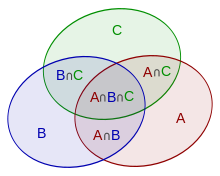# Inclusion-Exclusion Principle

### Introduction:

The inclusion-exclusion principle states that to count the unique ways of performing a task, we should add the number of ways to do it in a single way and the number of ways to do it in another way and then subtract the number of ways to do the task that is common to both the sets of ways.

In general, if there are, let’s say, 'N' sets, then the unique ways of performing the task are to add them separately and then subtract their pairwise intersection and then add the intersection of triples, and so on.

The inclusion-exclusion principle is also known as the subtraction principle. For any two sets with the total ways to do them be A1 and A2. Then the total unique ways to do both of them would be:-

| A1 ∪ A2 |= |A1 |+ | A2| – |A1 ∩ A2|

Let us try to understand it using the Venn Diagram:-Source: cp-algorithms.com

In the above picture, A, B, C are the three sets.

So, the union of all the three sets can be written as follows:

S(A∪B∪C)=S(A) + S(B) + S(C) − S(A∩B) − S(A∩C) − S(B∩C) + S(A∩B∩C)

Let us see few examples:-

### Example 1:

You need to find the total numbers less than 1000 divisible by both 2 and 7.

According to the definition of the Inclusion-Exclusion Principle, you will find the total numbers divisible by 2 and then total numbers divisible by 7, and then total numbers divisible by both 2 and 7.

Numbers divisible by 2 less than 1000, => 500

Numbers divisible by 7 less than 1000, => 142

Numbers divisible by both 2 and 7 less than 1000, => 71

Hence, unique numbers divisible by either 2 or 7 less than 1000 are

= 500 + 142 - 71 i.e., 571

### Example 2:

You need to count the number of Binary Strings of length 6, which start with “1” or ends with “11”.

For the Binary Strings starting with “1”, there are only 5 positions left. So, total Binary Strings starting with '1' are 2⁵, i.e., 32.

For the Binary Strings ending with “11”, there are only 4 positions left. So, total Binary Strings ending with “11” are 2⁴, i.e., 16.

For the Binary Strings starting with “1” and ending with “11”, there are only 3 positions left. So, total Binary Strings are 2³, i.e., 8.

So, the total number of Binary Strings starting with “1” or ending with “11” are 32 + 16 - 8, i.e., 40.

Now we will code our examples:

### Code 1:

#### Time Complexity:

Above code works in O(1) time complexity because we are not using any loop or recursion.

#### Space Complexity:

Above code works in O(1) space complexity because we are not using any auxiliary space.

## Code 2:

#### Time Complexity:

Above code works in O(1) time complexity because we are not using any loop or recursion.

#### Space Complexity:

Above code works in O(1) space complexity because we are not using any auxiliary space.

### FAQs:

How can we apply the inclusion-exclusion principle on N number of sets?

• First, add all the sets individually, then subtract them pair-wise, then add their triplets, and so on.

What is another name for the inclusion-exclusion principle?

• It is also known as the Subtraction principle.

### Key TakeAways:

In this blog, we have covered the following things:

1. What does the inclusion-exclusion principle state?
2. We saw a few examples of the inclusion-exclusion principle.
3. We wrote the code for them.

If you want to learn more about Data Structures and Algorithms and practice some questions requiring you to take your basic knowledge on Data Structures and Algorithms a notch higher, you can visit our Guided Path for DSA.

Until then, All the best for your future endeavors, and Keep Coding.BY: NISHANT RANA